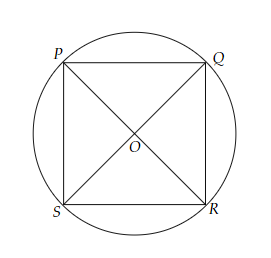# If diagonals of a cyclic quadrilateral are diameters of the circle through the vertices of the quadrilateral, prove that it is a rectangle.

Given:

The diagonals of a cyclic quadrilateral are diameters of the circle through the vertices of the quadrilateral.

To do:

We have to prove that it is a rectangle.

Solution:Let $PR$ and $QS$ be the diagonals of a cyclic quadrilateral $PQRS$.

This implies,

$PR$ and $QS$ are diameters of the circle.

$PR=QS$

$OP=OQ=OR=OS$    (Radii of the circle)

The diagonals of the quadrilateral $PQRS$ are equal and bisect each other.

Therefore, quadrilateral $PQRS$ is a rectangle.

Hence proved.

Updated on: 10-Oct-2022

81 Views